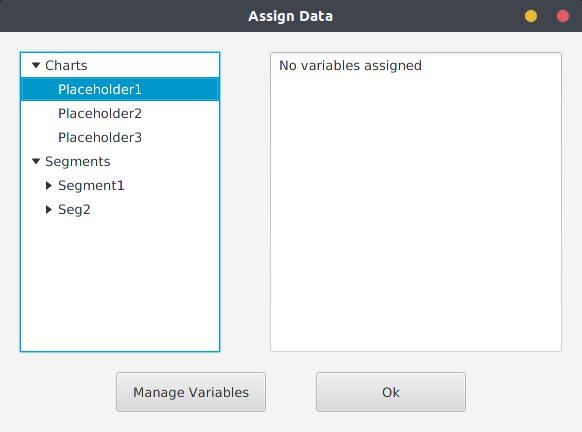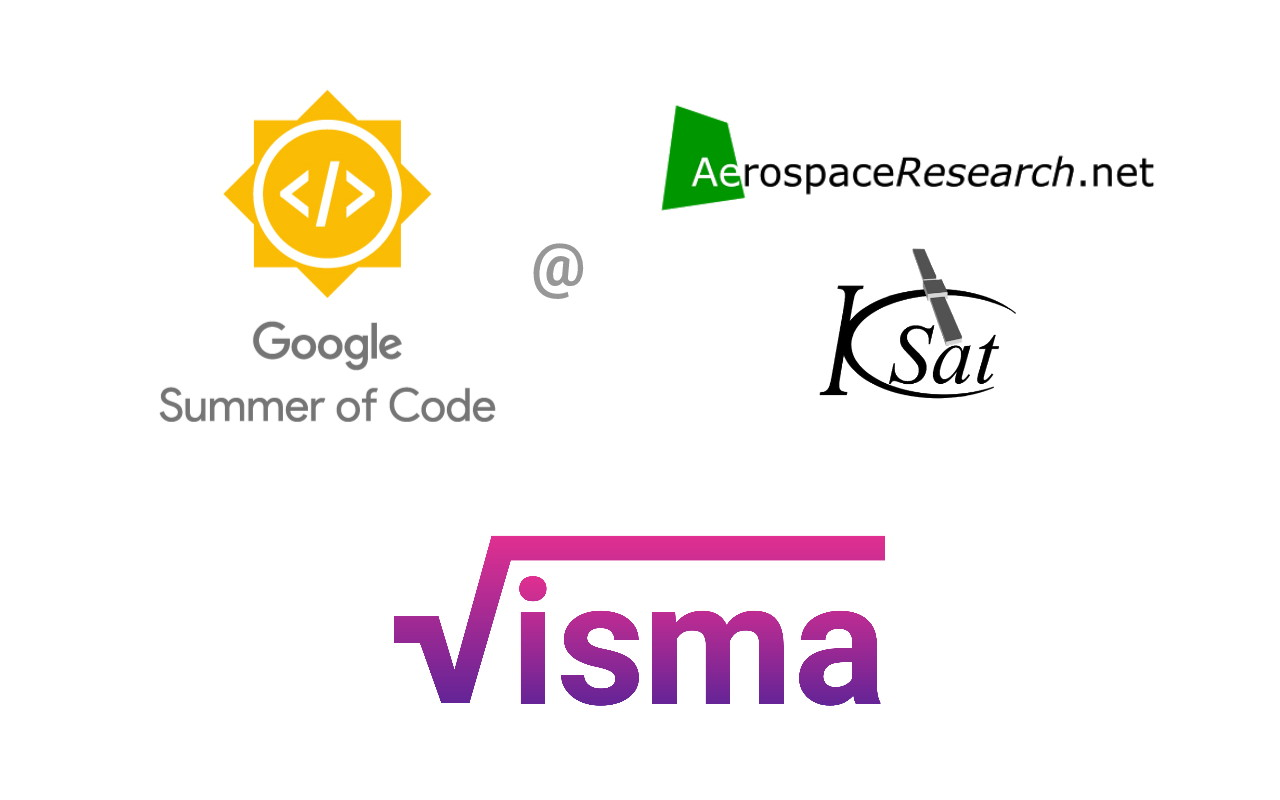## [GSoC2018|USOC|Pedro] Final Evaluations – My 3 month stay at AerospaceResearch

After 3 months of work towards AerospaceResearch, the time has come to write my last devblog related to this year’s edition of GSoC.  This might be a long one, so please bear with me for the next few minutes. At the end of the post, there’s a section with all my work throughout the project.

## Assigning data to UI elements

Since the last post, the AssignData Window has suffered considerable changes. I had to re-design it from scratch due to some limitations related to JavaFX’s TreeView implementation. Moving items from one tree to another causes them to behave incorrectly. I had to stick to another solution that may not look as smooth and intuitive but performs way better and actually works.

This is what it looks like:## [GSoC2018|DirectDemod|Vinay] Week #9-10: Accurate Meteor M2 and Funcube Sync detection

In the last blog we discussed how we were able to get the constallation and thus the bits from the input file. In this blog we will look at how we are accurately obtaining the sync positions within the file.

## [GSoC’18|VisMa|Shantanu] Week #10-11: Dynamic Simplification and Plotting

This is GSoC’18 log#05. Here I will cover on what I have done in week #10-11. Link to the previous log. The work during this period is mostly done in visma/gui.## Done so far…

A quick solver (visma/gui/qsolver.py) has been implemented which dynamically simplifies the expression as the user inputs. Instead of implementing the logger which was supposed to report if the input expression is invalid, I have implemented this feature in the qsolver itself. It lets the user know what is missing in the input or if the input syntax is wrong.

## [GSoC2018|OrbitDeterminator|Jorge] Week #7-8 – Refining Gauss method implementation

During these weeks, I worked on coding a high-level interface for two Gauss method applications of orbit determination: Earth satellites and asteroids.
One of the main differences with respect to my previous work, is that we relied heavily on the `astropy` library (www.astropy.org), a Python library for astronomical calculations. One of the main factors which inclined us to use this library, is that it has a lot of useful functions, it is relatively well-tested, gives more consistency to the code, and also avoids typos (e.g., in numerical values of astronomical constants). We also incorporated the `poliastro` library, mainly to use its implementation of the Stumpff functions.

### Changes in implementation

One of the main changes with respect to the previous version of our implementation, was the abstraction of a „core“ computation of the Gauss method and its refinement iterative stage, regardless of the case being handled, whether it is an Earth-orbiting object (satellite, space debris, etc.) or a Sun-orbiting object (main-belt asteroid, Near-Earth asteroid, comet, etc.). This abstraction is implemented in the `gauss_method_core` and `gauss_refinement` function, which are low level functions and may be adapted for user-defined applications.
A new feature of the latest version of the code, is that in case of having multiple solutions to the Gauss polynomial, now the user is able to select distinct roots for each occurrence of the multiple solutions. As we will see in examples below, multiple positive solutions to the Gauss polynomial are common when handling real-world data. In the case of finding more than one feasible solution to the Gauss polynomial, the code spits out all the feasible roots found; this allow the user to select the adequate root to the polynomial.
Also, the execution speed was improved for the Near-Earth asteroids case: when handling multiple observations, instead of reading the MPC-formatted file each time and retrieving the relevant triplet of observations, now the file is read only once. Since the observation files from MPC are typically a few thousand lines long, this improved the execution speeds about 10x.
Another important change, is that now the local mean sidereal time is computed using `astropy` ’s `sidereal_time` method for `SkyCoord` objects instead of using our own implementation. While doing this, we were able to identify a bug in our implementation of the computation of the local sidereal time!
Finally, in the case of Sun-orbiting bodies, the average orbital elements are computed in the heliocentric J2000.0 ecliptic frame; this allows us to directly compare our results, for example, with the orbital elements listed in the JPL’s Horizons online ephemeris service.

## [GSoC’18|OrbitDeterminator|Aakash] Week #7-8 : Propagation Model

`About the Author: Hello guys, I am Aakash Deep. I am an undergraduate student from Indraprastha Institute of Information Technology, Delhi (IIIT-D) in Computer Science and Engineering (CSE). I am a speed-cuber and, my hobby is solving Rubik's Cube. I am also founder of Rubik's Cube Club at IIIT-D.`

The post is in the continuation of the series of posts from the past. To read those blogs use these links for blog1, blog2 and blog3.

## Highlights

• The database module was added earlier to create and maintain/update the database. For the database, we used MySQL.
• The Gibb’s Method was implemented and OOP concepts were used for the ease of use and better maintenance.
• Propagation Model was implemented and the output was making some sense.

## [GSoC2018|Orbitdeterminator|Arya] Week #8-9: Implementing Kalman Filter

After the implementation of the Cowell propagator, it was time for the Kalman Filter. It is an algorithm that uses a series of measurements observed over time, containing statistical noise and other inaccuracies, and produces estimates of unknown variables that tend to be more accurate than those based on a single measurement alone. A Kalman Filter is the ideal filter for a linear process having Gaussian noise. Since our process is not linear, a modified version of the filter must be used. This is called the Extended Kalman Filter. It linearizes about the point in question and applies the regular Kalman Filter to it.

To apply the filter, the following things are required:

• A function f which outputs the next state, given the current state.
• The matrix F which is the jacobian of f .
• The matrix H which converts state into corresponding observations.
• A matrix Q denoting process noise. It consists of the inaccuracies in the model.
• A matrix R denoting measurement noise.

The variables involved during the calculations are:

• x – the state of the system
• z – the observations of the system
• P – covariance matrix of x. It denotes the inaccuracy in the estimate. As the filter runs, P is supposed to decrease.
• K – the Kalman gain. This matrix is between 0 and I and denotes how much the observations can be trusted vs how much the model can be trusted.

The filter consists of two major steps:

• Predict – in this step, we predict the next state (x) and covariance matrix (P) using our model.
• Update – in this step, we update our estimates of x and P using observations (z).

## [GSoC2018|OrbitDeterminator|Arya] Week #7-8: Implementing Cowell propagator

Last time when we tried to use the SGP4 propagator in our Kalman Filter, we ran into problems. This was because you can’t stop the algorithm at a certain stage and continue from there. Suppose you want to propagate from to , you can directly propagate from to but you can’t propagate it from to to . So I set out to make our own propagator taking into account two major perturbations – oblateness of Earth and atmospheric drag. Instead of making an analytical propagator like SGP4, I went with a numerical one because that can be stopped and started at any point. So the first challenge was making a numerical integrator.

### Numerical Integration

Numerical integration are used to integrate a differential equation from one point to another point. I studied and tested various integrators and finally settled on Runge-Kutta 4th order integrator (RK4).

• Good accuracy for small to medium step sizes
• Low computation cost
• Easy to implement

Let the initial value problem be and .

Choose a step size and define

for  where,

Note that these equations work equally well whether y is a scalar or a vector. In our case, the differential equation is . It is not in a form suitable to be used directly in RK4. To use it in RK4 we have to vectorize the equation. First, let’s define the state of the system as . Let . Then,This form can be directly used in the RK4 integrator.

## [GSoC’18|VisMa|Shantanu] Week #08-09: Develop, Test, Repeat

This is GSoC log#04 (view log#03 here). Here I will cover on what I have done in week #08-09 and a gist of what has been accomplished in Phase II coding period and what is to be done in the final coding period.## Done so far…

• Code coverage using coverage.py through pytest
• Created an equation solver in multi-variables
• Initialized work on matrices
• Ported source to Python3 and using PyQt5

## [GSoC’18|OrbitDeterminator|Aakash] Week #5-6 : Implementation of Gibb’s Method

`About the Author: Hello guys, I am Aakash Deep. I am an undergraduate student from Indraprastha Institute of Information Technology, Delhi (IIIT-D) in Computer Science and Engineering (CSE). I am a speed-cuber and, my hobby is solving Rubik's Cube.`

The post is in the continuation of the series of posts from the past. You can access those post in the following links, blog1 and blog2.

## Highlights

• The database was created for this, a code was written initDatabase.py. The code is responsible for creating a database with all the necessary tables in the database.
• The database is maintained by the script scraper.py. The script updates tables of the database.
• The propagation model is implemented. For this, the SGP4 algorithm is used. The algorithm is computing state vectors (a pair of position vector and velocity vector) from the TLE.

## Week 5 and 6

The Gibb’s method is implemented. It takes three position vectors as input and after computation gives one position vector and one velocity vector (both as combined also known as a state vector) as output. The OOP concepts are used in the implementation of Gibb’s method. The code is in gibbsMethod.py. By using OOP concepts it supports data abstraction and data encapsulation.

For input, a file is used from /example_data. It contains four attributes which are time, x coordinate, y coordinate and z coordinate. These are the position coordinates at that particular time epoch. As the file contains a lot of position vectors (8000 to be precise), a set of consecutive 3 vectors are used at a time for gibb’s method. Then for the next iteration, the first vector is removed and a new third vector is added to the set. As we are computing state vectors from a set of three vectors, it is obvious that the number of output state vectors is two less than the input position vectors. So, a vector is created by taking the previous length into consideration which holds all the output state vectors. The class also contains a function orbital_elements which converts these state vectors into the orbital elements.

The propagation model, SGP4 is also improved. Previously, the output is not making much sense but after revising it now the velocity vector is making sense. The output of the position vector is coming out to be constant for all the output state vector. Working on position vector in order to get a good output.

### Work in Progress

• Documentation
• Testing

## [GSoC2018|DirectDemod|Vinay] Week #7-8: Meteor M2 demodulation (QPSK) and BPSK demodulation

Many modern communication systems are moving away from traditional analog communication methods to digital communication systems. Using a digital system makes the communication more robust, however will make the system complicated. In this blog we will look at the PSK (Phase shift keying) modulation, specifically QPSK and BPSK.